## Big babies: The National Health Statistics Reports described a study in which a sample of 315 one-year-old baby boys were weighed. Their mea

Question

Big babies: The National Health Statistics Reports described a study in which a sample of 315 one-year-old baby boys were weighed. Their mean weight was 25.6 pounds with standard deviation 5.3 pounds. A pediatrician claims that the mean weight of one-year-old boys is greater than 25 pounds. Do the data provide convincing evidence that the pediatrician’s claim is true? Use the =α0.05 level of significance and the critical value method with the

in progress 0
5 months 2021-08-18T17:16:48+00:00 1 Answers 12 views 0

The pvalue of the test is 0.0012 < 0.05, which means that the data provides convincing evidence that the pediatrician’s claim is true.

Step-by-step explanation:

A pediatrician claims that the mean weight of one-year-old boys is greater than 25 pounds.

This means that at the null hypothesis, we test that the mean is 25 pounds, that is: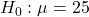At the alternate hypothesis, we test that it is more than 25 pounds, that is: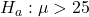The test statistic is: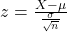In which X is the sample mean,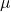is the value tested at the null hypothesis,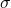is the standard deviation and n is the size of the sample.

25 is tested at the null hypothesis:

This means that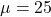The National Health Statistics Reports described a study in which a sample of 315 one-year-old baby boys were weighed. Their mean weight was 25.6 pounds with standard deviation 5.3 pounds.

This means that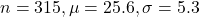Value of the test-statistic: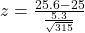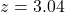Pvalue of the test and decision:

The pvalue of the test is the probability of finding a mean above 25.6 pounds, which is 1 subtracred by the pvalue of z = 3.04.

Looking at the z-table, z = 3.04 has a pvalue of 0.9988

1 – 0.9988 = 0.0012

The pvalue of the test is 0.0012 < 0.05, which means that the data provides convincing evidence that the pediatrician’s claim is true.